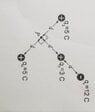# Find the Electric field at point p

• NotInMrPutmansClass

#### NotInMrPutmansClass

Homework Statement
Calculate the net electric field at point P from the diagram below
Relevant Equations
E=(kq)/r^2

Where k is plank's constant 9×10^9
Since q3=q4 and they are opposite to each others they cancel out
But as soon as I try to find the electric field of one of the charges, I need the radius which is not given.

By isolating the electric field for radius

E=(kq)/r^2
I now have two unknowns

#### Attachments

•20221115_073652.jpg
17.2 KB · Views: 40
Can you post the full problem statement as it was given? (k is Coulomb's constant, not Planck's constant.)

•topsquark
Homework Statement:: Calculate the net electric field at point P from the diagram below
Relevant Equations:: E=(kq)/r^2

Where k is plank's constant 9×10^9
•